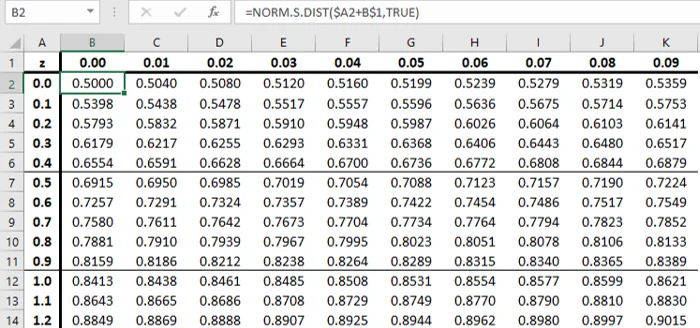Super learner

09 Mar 2023

Posted on:

06 Mar 2023

0

# Resolved:How to make a z-table?

We were given a z-table in the Course notes and it helped with Tasks 2 & 3 of the exercise, but what if we didn't have one? How would we make it? I have to admit I had to study the solution file very closely to understand what the correct answer was, and I had to watch the lesson multiple times . I did get the right answer for Tasks 2 & 3 eventually!

Super learner
Posted on:

08 Mar 2023

2

Hi Dylan,

This is what I have done (as attached at the bottom of the message).

In the first row I put 0.00, 0.01, ..., 0.09 and in the first column I put 0.0, 0.1, 0.2, ..., 3.5.  In Cell B2, I input the formula "=NORM.S.DIST(\$A2+B\$1,TRUE)".  Afterwards, drag the formula to the rest of the table and the z-table is completed.

Due to the symmetry property of the standard normal, I only include the positive z-value.

Hope this helpsSuper learner
Posted on:

08 Mar 2023

0

Thank you for this detailed answer! This may be an obvious answer but is there a reason you stopped at 0.09 and 3.5 respectively? Could you make the row extend to 1 and column to 15 for example? I don't understand the row or column limits of the z-table.

Super learner
Posted on:

09 Mar 2023

1

The column value (0.00, 0.01,..., 0.09) refers to the 2nd decimals of the z value.  For example, if z = 1.23, I will look for the row with value 1.2 and the column with value 0.03.  Hence, the value of P(Z<1.23) = 0.8907.  Of course , we can directly ask Excel to compute the exact value (I.e. =NORM.S.DIST(1.23, TRUE) ) instead of using the table.

Regarding the row extending to 15, there is no need to do this because when z is greater than 3.5 or even 4, the value in the table is very close to 1.

Super learner
Posted on:

09 Mar 2023

1

Thank you for this useful information and explanations Eugene!